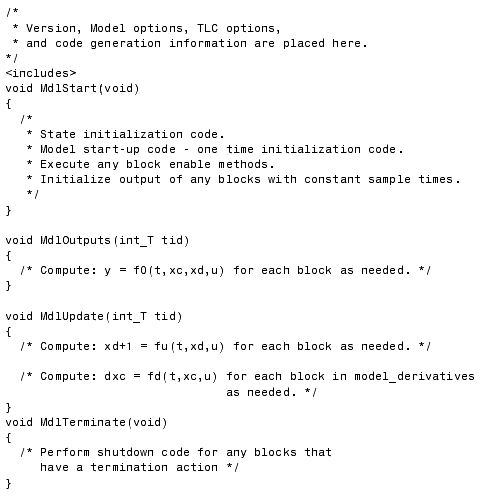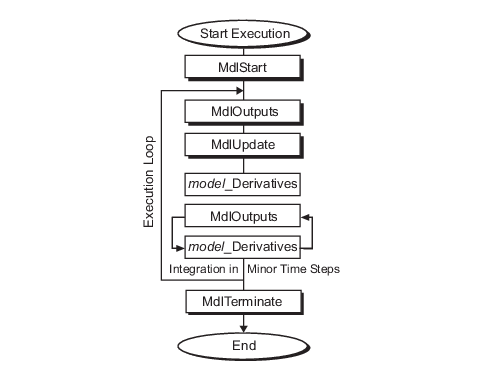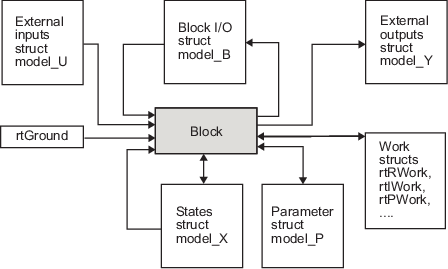## Rapid Prototyping Model Functions

Rapid prototyping code defines the following functions that interface with the main program (`main.c` or `main.cpp`):

• `Model()`: The model registration function. This function initializes the work areas (for example, allocating and setting pointers to various data structures) used by the model. The model registration function calls the `MdlInitializeSizes` and `MdlInitializeSampleTimes` functions. These two functions are very similar to the S-function `mdlInitializeSizes` and `mdlInitializeSampleTimes` methods.

• `MdlStart(void)`: After the model registration functions `MdlInitializeSizes` and `MdlInitializeSampleTimes` execute, the main program starts execution by calling `MdlStart`. This routine is called once at startup.

The function `MdlStart` has four basic sections:

• Code to initialize the states for each block in the root model that has states. A subroutine call is made to the “initialize states” routines of conditionally executed subsystems.

• Code generated by the one-time initialization (start) function for each block in the model.

• Code to enable the blocks in the root model that have enable methods, and the blocks inside triggered or function-call subsystems residing in the root model. Simulink® blocks can have enable and disable methods. An enable method is called just before a block starts executing, and the disable method is called just after the block stops executing.

• Code for each block in the model whose output value is constant. The block code appears in the `MdlStart` function only if the block parameters are not tunable in the generated code and if the code generator cannot eliminate the block code through constant folding.

• `MdlOutputs(int_T tid)`: `MdlOutputs` updates the output of blocks. The `tid` (task identifier) parameter identifies the task that in turn maps when to execute blocks based upon their sample time. This routine is invoked by the main program during major and minor time steps. The major time steps are when the main program is taking an actual time step (that is, it is time to execute a specific task). If your model contains continuous states, the minor time steps will be taken. The minor time steps are when the solver is generating integration stages, which are points between major outputs. These integration stages are used to compute the derivatives used in advancing the continuous states.

• `MdlUpdate(int_T tid)`: `MdlUpdate` updates the states and work vector state information (that is, states that are neither continuous nor discrete) saved in work vectors. The `tid` (task identifier) parameter identifies the task that in turn indicates which sample times are active, allowing you to conditionally update only states of active blocks. This routine is invoked by the interface after the major `MdlOutputs` has been executed. The solver is also called, and `model_Derivatives` is called in minor steps by the solver during its integration stages. All blocks that have continuous states have an identical number of derivatives. These blocks are required to compute the derivatives so that the solvers can integrate the states.

• `MdlTerminate(void)`: `MdlTerminate` contains any block shutdown code. `MdlTerminate` is called by the interface, as part of the termination of the real-time program.

The contents of the above functions are directly related to the blocks in your model. A Simulink block can be generalized to the following set of equations.

`$y={f}_{0}\left(t,{x}_{c},{x}_{d},u\right)$`

Output y is a function of continuous state xc, discrete state xd, and input u. Each block writes its specific equation in a section of `MdlOutputs`.

`${x}_{d+1}={f}_{u}\left(t,{x}_{d},u\right)$`

The discrete states xd are a function of the current state and input. Each block that has a discrete state updates its state in `MdlUpdate`.

`$\stackrel{˙}{x}={f}_{d}\left(t,{x}_{c},u\right)$`

The derivatives x are a function of the current input. Each block that has continuous states provides its derivatives to the solver (for example, `ode5`) in `model_Derivatives`. The derivatives are used by the solver to integrate the continuous state to produce the next value.

The output, y, is generally written to the block I/O structure. Root-level Outport blocks write to the external outputs structure. The continuous and discrete states are stored in the states structure. The input, u, can originate from another block's output, which is located in the block I/O structure, an external input (located in the external inputs structure), or a state. These structures are defined in the `model.h` file that the Simulink Coder™ software generates.

The next example shows the general contents of the rapid prototyping style of C code written to the `model.c` file.This figure shows a flow chart describing the execution of the rapid prototyping generated code.

Rapid Prototyping Execution Flow ChartEach block places code in specific `Mdl` routines according to the algorithm that it is implementing. Blocks have input, output, parameters, and states, as well as other general items. For example, in general, block inputs and outputs are written to a block I/O structure (`model_B`). Block inputs can also come from the external input structure (`model_U`) or the state structure when connected to a state port of an integrator (`model_X`), or ground (`rtGround`) if unconnected or grounded. Block outputs can also go to the external output structure (`model_Y`). This figure shows the general mapping between these items.

Data View of the Generated CodeThe following list defines the structures shown in the preceding figure:

• Block I/O structure (`model_B`): This structure consists of persistent block output signals. The number of block output signals is the sum of the widths of the data output ports of the nonvirtual blocks in your model. If you activate block I/O optimizations, the Simulink and Simulink Coder products reduce the size of the `model_B` structure by

• Reusing the entries in the `model_B` structure

• Making other entries local variables

See How Generated Code Stores Internal Signal, State, and Parameter Data for more information on these optimizations.

Structure field names are determined either by the block's output signal name (when present) or by the block name and port number when the output signal is left unlabeled.

• Block states structures: The continuous states structure (`model_X`) contains the continuous state information for blocks in your model that have continuous states. Discrete states are stored in a data structure called the ```DWork vector (model_DWork)```.

• Block parameters structure (`model_P`): The parameters structure contains block parameters that can be changed during execution (for example, the parameter of a Gain block).

• External inputs structure (`model_U`): The external inputs structure consists of the root-level Inport block signals. Field names are determined by either the block's output signal name, when present, or by the Inport block's name when the output signal is left unlabeled.

• External outputs structure (`model_Y`): The external outputs structure consists of the root-level Outport blocks. Field names are determined by the root-level Outport block names in your model.

• Real work, integer work, and pointer work structures (`model_RWork`, `model_IWork`, `model_PWork`): Blocks might have a need for real, integer, or pointer work areas. For example, the Memory block uses a real work element for each signal. These areas are used to save internal states or similar information.